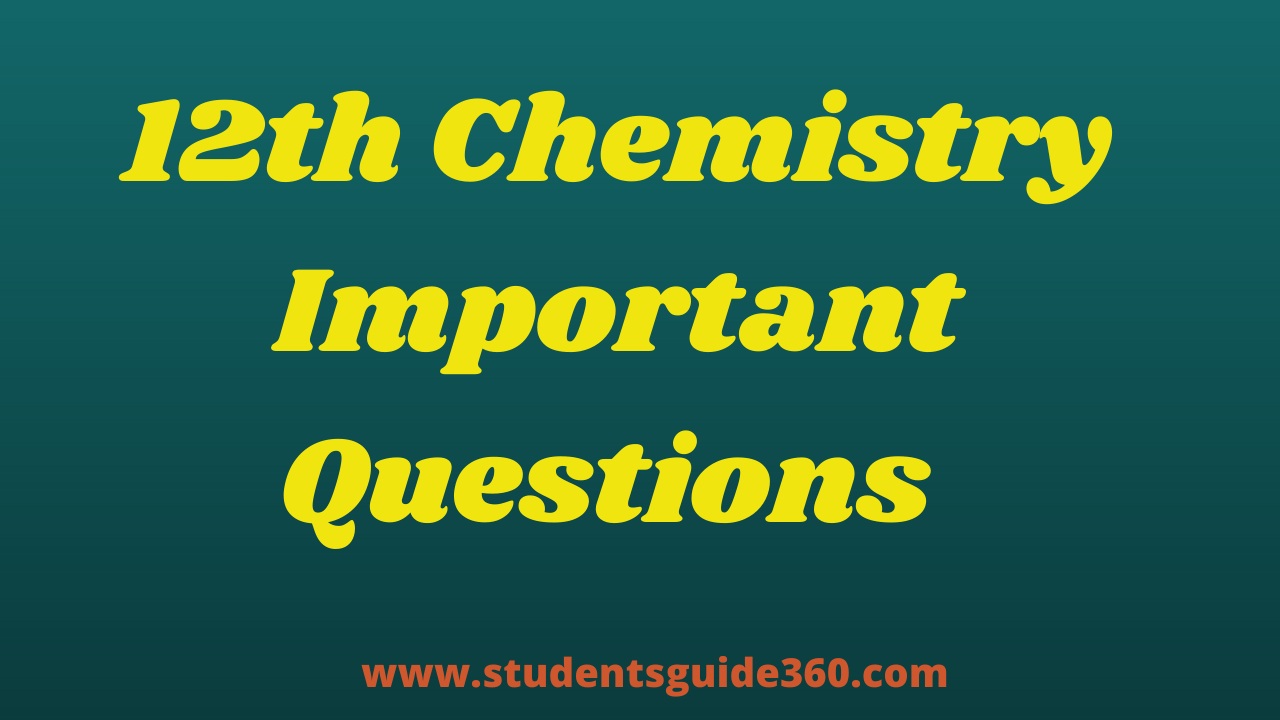# 12th Chemistry Important 1 Marks Ionic – equilibrium

## One Mark Study Material Unit wise as per reduced syllabus (2021-22)

TN 12th Chemistry Important 1 Marks Ionic – equilibrium. 12th Chemistry Important Questions Chapter 8. +2 Chemistry Important 2 Marks,  3 Marks, 5 Marks Questions Based on reduced Syllabus 2021-2022. 12th Free Online Test (MCQs). HSC 12th Chemistry Revision Test Important Questions. 12th Tamil Full Guide.

## Ionic – equilibrium

• 1. Dissociation constant of ammonium hydroxide(NH4OH) is 1.8 x 10-5 the hydrolysis constant of ammonium chloride (NH4Cl) would be 5.55 x 10-10
• 2. Calculate the pH of 0.04 M HNO3 solution. pH = 1.40
• 3. The concentration of hydroxide ion in a water sample is found to be 2.5 x 10-6M . What is the nature of the solution? pH solution is basic (pH =14 – 5.6021) =8.3979.
• 4. A lab assistant prepared a solution by adding a calculated quantity of Hcl gas at 250c to get a solution with[H3O+] = 4×10-5M. Is the solution neutral(or) acidic(or) basic. pH solution is acidic. (pH = 4.398)
• 5. The hydrogen ion concentration of a buffer solution consisting of a weak acid and its salt is given by [H+] = Ka [acid]/[salt]
• 6. 𝐡 = √ Kw/Ka.Kb this relation is correct for degree of hydrolysis of ammonium acetate.
• 7. The pH of an aqueous solution is zero. The solution is strongly acidic
• 8. HPO42- can act as Lowery-Bronsted acid as well as base.
• 9. H2PO42- the conjugate base of H3PO4
• 10. The pH of 10-5 M KOH solution will be 9
• 11. The dissociation constant of weak acid is 1×10-3. In order to prepare a buffer solution. With a pH =4, the [Acid]/[Salt] ratio should be 1:10
• 12. The % of pyridine that forms pyridinium ion in a 0.10M aqueous pyridine solution is
0.013%
• 13. Equal volumes of 3 acid solutions of pH 1,2,& 3 are mixed in a vessel. What will be the H+ ion concentration in the mixture? 3.7 x 10-2
• 14. The solubility of Agcl(s) with solubility product 1.6 x 10-10 in 0.1M Nacl solution would be 1.6 x 10-9M
• 15. If solubility product of lead iodide is 3.2 x 10-8, it solubility will be 2×10-3M
• 16. MY & NY3, are insoluble salts, and have the same Ksp values of6.2 x 10-13 at room temperature. Which statement would be true with regard to My & NY3? The molar solubility of MY in water is less than that of NY3
• 17. What is the pH of the resulting solution when equal volumes of 0.1M NaOH and 0.01M Hcl are mixed? 12.65
• 18. The aqueous solution of sodium formate, anilinium chloride and potassium cyanide are respectively is basic, acidic, basic.
• 19. BF3 is not lkely to act as lewis acid.
• 20.PF3 is most likely to behave as a lewis base.
• 21. 100ml of 0.1M Hcl + 200 ml of 0.1 M NH4OH it act as basic buffer.
• 22. Conjugate base for bronsted acids H2O & HF are OH & F respectively.
• 23. pH of a saturated solutions of Ca(OH)2 is 9 . The solubility product(Ksp) of Ca(OH)2 is
0.5 x 10-15
• 24. The solubility of BaSO4 in water is 2.42 x 10-3gL-1 at 298K The value of its solubility product will be. (BaSO4 = 233g.mol-1) 1.08 x 10-10 mol2L-2.
• 25. 75ml M/5 HCl + 25ml M/5 NaOH solutions were prepared by mixing different volumes of NaOH of Hcl different concentrations.
• 26. Concentration of the Ag+ ions in a saturated solution of Ag2C2O4 is 2.24 x 10-4 mol.L-1 solubility product of Ag2C2O4 is 5.619 x 10-12 mol3L-3
• 27. Lewis acid example : BF3, AlCl3, BeF2.
• 28. Lewis Base example : NH3,R-NH2, F, Cl
• 29. pH = – log10[H3O+]
• 30.pOH = -log10[OH]
• 31. Kb for NH4OH is 1.8 x 10-5. Calculate the percentage of ionization of 0.06 M ammonium hydroxide solution is Formula 𝛼 =√Kb/C 1.732%
• 32. Lewis acid – Carbonium ion
• 33. Lowry bronsted theory – Has a tendency to donate proton
• 34. Calculate the pH of 0.1 M CH3COONa solution (pKa for CH3COOH is 4.74). is formula pH = 7 + pKa/2 + log C/2 8.87
• 35. Arrhenius concept is Gives H+ in water.
• 36. Ostwald’s dilution law is Ka = 𝜶2C
• 37. pH Value of Vinegar : 2
• 38. pH value of Black coffee is 5
• 39. pH value of Baking soda is 9
• 40 pH value of Soapy water is 12